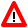# Performance Considerations

This document covers the following topics:

## Formats

Best performance is achieved when you use the data formats packed numeric (P) and integer (I4) in arithmetic operations.

Avoid converting data between the formats packed numeric (P), unpacked numeric (N), integer (I), and floating point (F), as this causes processing overhead even with optimized code.

As there is no interpretation overhead with optimized code, the differences between the various data formats become much more prominent: with optimized code the performance improvement gained by using format P instead of N, for example, is even higher than with normal code.

#### Example:

`A = A + 1`

In the above numeric calculation

• with non-optimized code, format P executes approximately 13 % faster than format N.

• with optimized code, however, format P executes approximately 56 % faster than format N.

The performance gain which would be achieved by applying the Natural Optimizer Compiler to this simple statement is

• with unpacked operands (N): 8 times faster

• with packed operands (P): 15 times faster

## Arrays

Array range operations, such as

`MOVE A(*) TO B(*)`

are executed more efficiently than if the same function were programmed using a `FOR` statement processing loop. This is also true for optimized code.

When indexes are used, integer format I4 should be used to achieve optimum performance.

## Alphanumeric Fields

We recommend that you adjust the length of the alphanumeric constant to the length of the variable, when moving an alphanumeric constant to an alphanumeric variable (format A), or when comparing an alphanumeric variable with an alphanumeric constant. This will significantly speed up operation, for example:

```A(A5):='XYZAB'

...
IF A = 'ABC  ' THEN ...```

is faster than

`IF A = 'ABC' THEN ...`

## DECIDE ON

When using the `DECIDE ON` statement with a system variable, array or parameter `operand1`, it is more efficient to move the value to a scalar variable of the same type and length defined in the `LOCAL` storage section.

## Numeric Values

When using numeric constants in assignments or arithmetic operations, try to force the constants to have the same type as the operation.

### Rules of Thumb

• Any numeric constant with or without a decimal but without an exponent is compiled to a packed number having the minimum length and precision to represent the value, unless the constant is an array index or substring starting position or length, in which case it becomes a four-byte integer (I4). This rule applies irrespective of the variable types participating in the operation.

• Operations containing floating point will be executed in floating point. Add `E00` to numeric values to force them to be floating point, for example:

`ADD 1E00 to F(F8)`
• Operations not containing floating point, but containing packed numeric, unpacked numeric, date or time variables will be executed in packed decimal. For `ADD`, `SUBTRACT` and `IF`, force numeric constants to have the same number of decimal places as the variable with the highest precision by adding a decimal place and trailing zeros, for example:

`ADD 1.00 TO P(P7.2)`

This technique is unnecessary for `MULTIPLY` and `DIVIDE`.

## Variable Positioning

To ease the optimization process, try to keep all scalar references at the front of the data section and all array references at the end of the data section.

## Variable Caching

The Natural Optimizer Compiler contains an algorithm to enhance the performance even further. In terms of performance, a statement will differ depending on the types of operands. The statement will execute more slowly if one or more of the operands is a parameter, array or scalar field of Type N (numeric) or combinations of these operands. The NOC analyzes the program flow and determines which variables with one or more of these characteristics are read two or more times without being written to. It then moves the value of each variable to a temporary cache area where it can be accessed quickly under the following conditions:

• The variable is accessed often but seldom modified and

• The variable is an array of any type or a scalar field of Type N (numeric).

Most suitable for variable caching are programs with long sequences that repeatedly access the same variable, in particular if the variable is an array. Variable caching then avoids complex and recurring address computation.

### Example of Variable Caching

The example program displayed below demonstrates the advantage of variable caching. Cataloged with `NODBG` (see below) and `CACHE=ON`, executing this program in a test environment took 47 % of the time required to execute the program with `NODBG` and `CACHE=OFF`. Cataloging the program with `CACHE=ON`, reduces the code generated by the NOC from 856 bytes to 376 bytes.

```DEFINE DATA LOCAL
1 ARR(N2/10,10,10)
1 I(I4) INIT <5>
1 J(I4) INIT <6>
1 K(I4) INIT <7>
END-DEFINE
DECIDE ON EVERY ARR(I,J,K)
VALUE 10 IGNORE
VALUE 20 IGNORE
VALUE 30 IGNORE
VALUE 40 IGNORE
VALUE 50 IGNORE
VALUE 60 IGNORE
VALUE 70 IGNORE
VALUE 80 IGNORE
VALUE 90 IGNORE
NONE IGNORE
END-DECIDE```Warning:If the content of a cached variable is modified with the command `MODIFY VARIABLE` of the Natural Debugger, only the content of the original variable is modified. The cached value (which may still be used in subsequent statements) remains unchanged. Therefore, variable caching should be used with great care if the Natural Debugger is used. See also the Natural Debugger documentation.

## NODBG

Once a program has been thoroughly tested and put into production, you should catalog the program with the `NODBG` option as described in the section Optimizer Options. Without debug code, the optimized statements will execute from 10% to 30% faster.

The code to facilitate debugging is removed when this option is specified, even with `INDX` or `OVFLW` options turned on.